# Chapter 3 Discrete Random Variables and Probability Distributions

• Slides: 15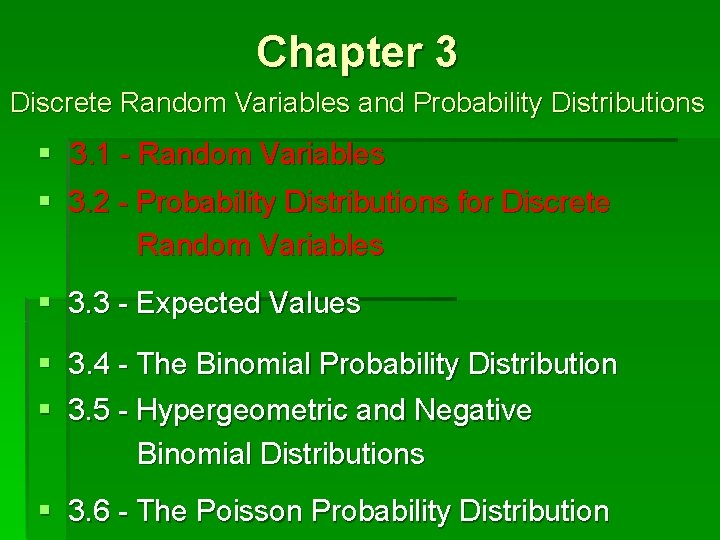Chapter 3 Discrete Random Variables and Probability Distributions § 3. 1 - Random Variables § 3. 2 - Probability Distributions for Discrete Random Variables § 3. 3 - Expected Values § 3. 4 - The Binomial Probability Distribution § 3. 5 - Hypergeometric and Negative Binomial Distributions § 3. 6 - The Poisson Probability Distribution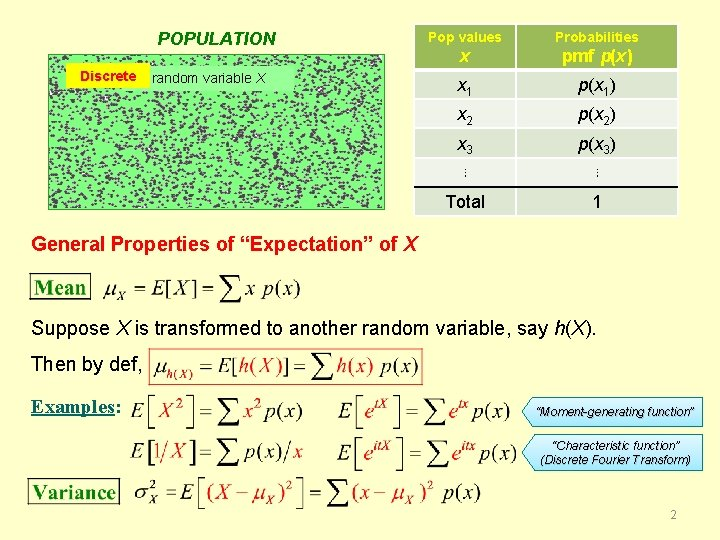POPULATION Discrete random variable X Pop values Probabilities x pmf p(x) x 1 p(x 1) x 2 p(x 2) x 3 p(x 3) ⋮ ⋮ Total 1 General Properties of “Expectation” of X Suppose X is transformed to another random variable, say h(X). Then by def, Examples: “Moment-generating function” “Characteristic function” (Discrete Fourier Transform) 2POPULATION Discrete random variable X Pop values Probabilities x pmf p(x) x 1 p(x 1) x 2 p(x 2) x 3 p(x 3) ⋮ ⋮ Total 1 General Properties of “Expectation” of X Suppose X is constant, say b, throughout entire population… Then by def, b 3POPULATION Discrete random variable X Pop values Probabilities x pmf p(x) x 1 p(x 1) x 2 p(x 2) x 3 p(x 3) ⋮ ⋮ Total 1 General Properties of “Expectation” of X Suppose X is constant, say b, throughout entire population… Then… 4POPULATION Discrete random variable X Pop values Probabilities x pmf p(x) x 1 p(x 1) x 2 p(x 2) x 3 p(x 3) ⋮ ⋮ Total 1 General Properties of “Expectation” of X Multiply X by any constant a… Then by def, a 5POPULATION Discrete random variable X Pop values Probabilities x pmf p(x) x 1 p(x 1) x 2 p(x 2) x 3 p(x 3) ⋮ ⋮ Total 1 General Properties of “Expectation” of X Multiply X by any constant a… Then… i. e. , … 6POPULATION Discrete random variable X Pop values Probabilities x pmf p(x) x 1 p(x 1) x 2 p(x 2) x 3 p(x 3) ⋮ ⋮ Total 1 General Properties of “Expectation” of X Multiply X by any constant a… Add any constant b to X… Then… i. e. , … 7POPULATION Discrete random variable X Pop values Probabilities x pmf p(x) x 1 p(x 1) x 2 p(x 2) x 3 p(x 3) ⋮ ⋮ Total 1 General Properties of “Expectation” of X Multiply X by any constant a… Add any constant b to X… Then… i. e. , … 8POPULATION Discrete random variable X Pop values Probabilities x pmf p(x) x 1 p(x 1) x 2 p(x 2) x 3 p(x 3) ⋮ ⋮ Total 1 General Properties of “Expectation” of X 9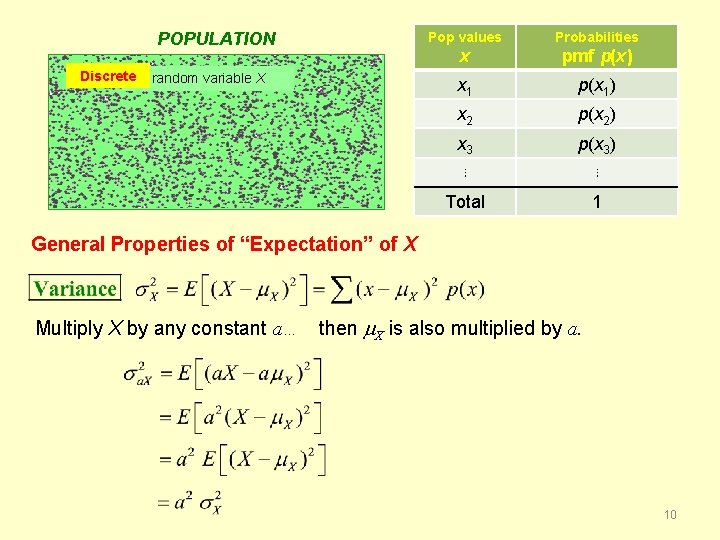POPULATION Discrete random variable X Pop values Probabilities x pmf p(x) x 1 p(x 1) x 2 p(x 2) x 3 p(x 3) ⋮ ⋮ Total 1 General Properties of “Expectation” of X Multiply X by any constant a… then X is also multiplied by a. 10POPULATION Discrete random variable X Pop values Probabilities x pmf p(x) x 1 p(x 1) x 2 p(x 2) x 3 p(x 3) ⋮ ⋮ Total 1 General Properties of “Expectation” of X Multiply X by any constant a… then X is also multiplied by a. i. e. , … 11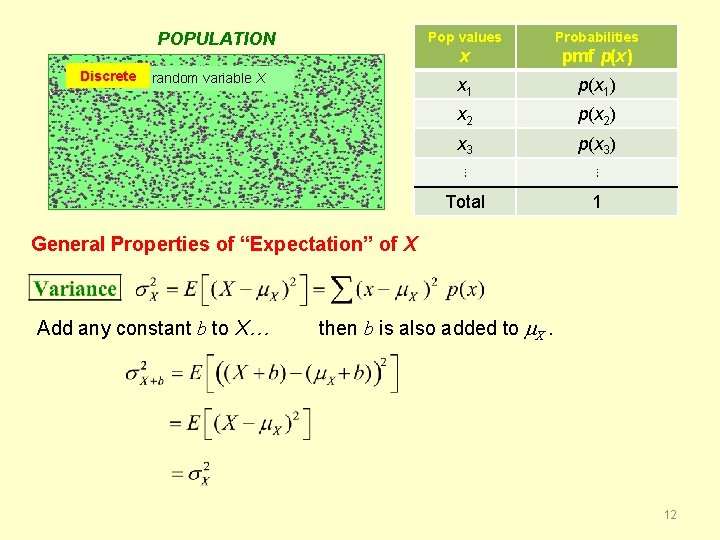POPULATION Discrete random variable X Pop values Probabilities x pmf p(x) x 1 p(x 1) x 2 p(x 2) x 3 p(x 3) ⋮ ⋮ Total 1 General Properties of “Expectation” of X Add any constant b to X… then b is also added to X. 12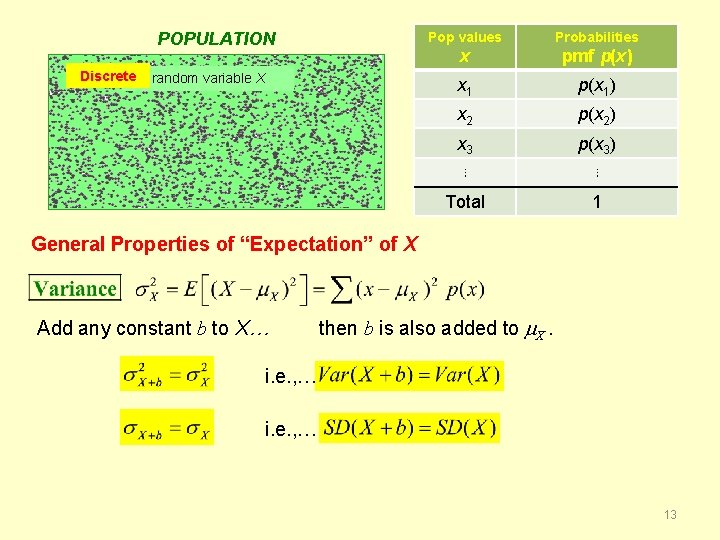POPULATION Discrete random variable X Pop values Probabilities x pmf p(x) x 1 p(x 1) x 2 p(x 2) x 3 p(x 3) ⋮ ⋮ Total 1 General Properties of “Expectation” of X Add any constant b to X… then b is also added to X. i. e. , … 13POPULATION Discrete random variable X Pop values Probabilities x pmf p(x) x 1 p(x 1) x 2 p(x 2) x 3 p(x 3) ⋮ ⋮ Total 1 General Properties of “Expectation” of X 14POPULATION Discrete random variable X Pop values Probabilities x pmf p(x) x 1 p(x 1) x 2 p(x 2) x 3 p(x 3) ⋮ ⋮ Total 1 General Properties of “Expectation” of X This is the analogue of the “alternate computational formula” for the sample variance s 2. 15